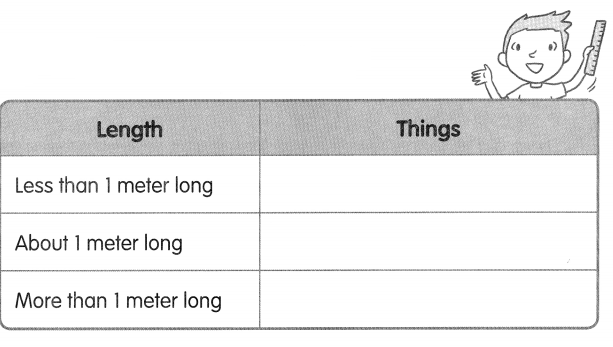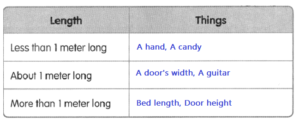# Math in Focus Grade 2 Chapter 7 Practice 1 Answer Key Measuring in Meters

Practice the problems of Math in Focus Grade 2 Workbook Answer Key Chapter 7 Practice 1 Measuring in Meters to score better marks in the exam.

## Math in Focus Grade 2 Chapter 7 Practice 1 Answer Key Measuring in Meters

Look at the pictures.

Fill in the blanks with more or less.

Question 1.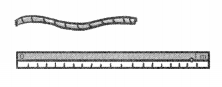The length of the rope ¡s _________ than 1 meter.
Answer: The length of the rope is less than 1 meter.

Question 2.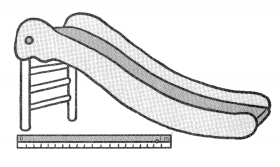The length of the slide is ___ than 1 meter.
Answer: The length of the slide is more than 1 meter.

Question 3.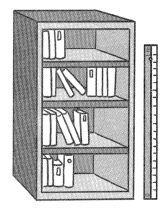The height of the bookcase is __ than 1 meter.
Answer: The height of the bookcase is more than 1 meter

Fill in the blanks.

Question 4.
Metersticks are placed against two boxes.
a. Which box is about 1 meter long? Box _____

Fill in the blanks with more or less.

b. Box A is ___ than 1 meter long.

c. Box B is ___ than 1 meter long.

Question 5.
Metersticks are placed against a board.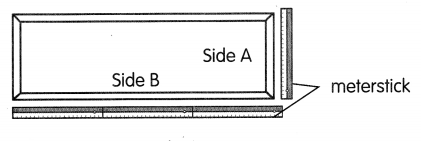a. Which side of the board is about 3 meters long? Side _____

b. Side A is shorter than ___ meter.
Answer: Side A is shorter than 1 meter.

c. Side B is shorter than ___ meters.
Answer: Side B is shorter than 3 meters.

Look at the list below.
Check (✓) the columns that are true.
You will need a meterstick or a 1-meter string to measure some items.

Question 6.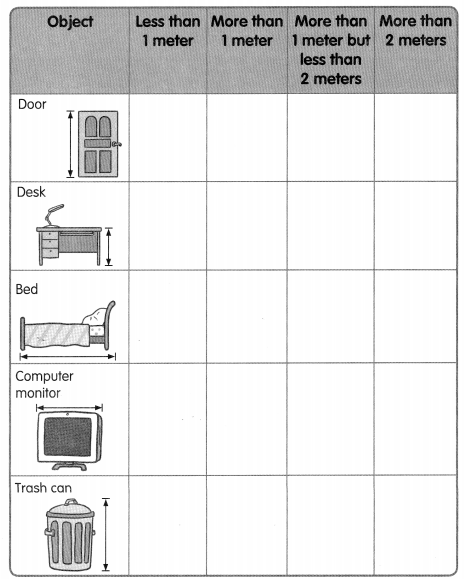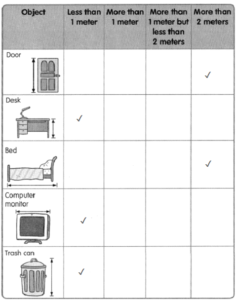Name three things that match each length.

Question 7.